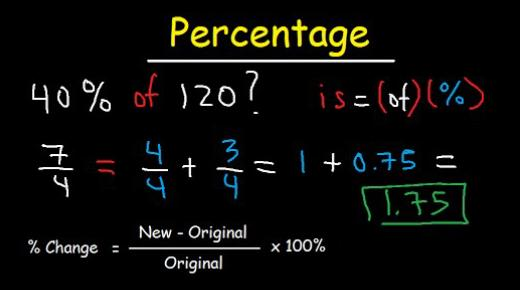# TABE: Can You Find The Percentage? Math Trivia Quiz

12 Questions | Total Attempts: 275SettingsAre you looking for a TABE math trivia quiz? Can you find the percentage? If you have been having a hard time when it comes to percentages, the quiz below is perfect for seeing how true that is and if you stand a chance of passing any assessment test brought to you with this type of math problems. Why don’t you give it a try and refresh your mind?

Related Topics
• 1.
90% of 80
• 2.
10% of 50
• 3.
138% of 10
• 4.
90% of 40
• 5.
30% of ___ =18
• 6.
190% of ___ =19
• 7.
90% of ___ = 72
• 8.
160% of ___ = 48
• 9.
____% of 110 =77
• 10.
___% of 100 =10
• 11.
____% of 90=36
• 12.
___% of 140=14